Next: Beamstrahlung Up: Laser Previous: Linear Compton Scattering

### Quantum Electrodynamics Involving a Strong Laser Field

When the laser field is strong, the simple formulas of Compton/Breit-Wheeler can nolonger be used. The laser strength is characterized by the parameter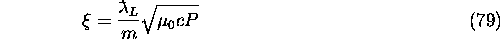where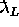is the laser wavelength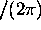in meter, m the electron rest mass in eV/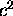, c the velocity of light in m/s,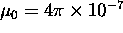, and P the power density in Watt/m. When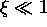, the simple formas are enough but as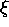becomes large, the probability of absorbing more than one photon in the laser field cannot be ignored. When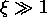, the constant-field approximationbecomes good. If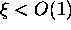, the expansion in terms of the number of absorbed photons, n, shows good convergence. The expansion takes a relatively simple form when the laser is circularly polarized by 100%. The present version accepts such case only.

In the case of the nonlinear Compton process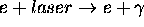, the number of emitted photons per unit time can be expanded in the form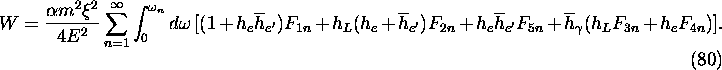E,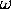Energy of the initial electron and final photon.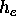,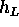Helicity of the initial electron and laser (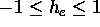,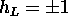).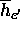,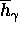`Detector helicity' of the final electron and photon.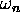Maximum photon energy when n laser photons are absorbed: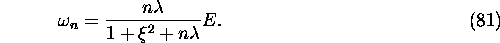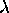Laser energy parameter: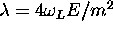. (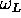: laser photon energy)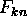Functions involving Bessel functions. See Sec.A.1 for the definition.
The photon emission angle is uniquely determined from n and.

The formula for the nonlinear Breit-Wheeler process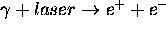can be written in a similar form. The total number of pair electrons per unit time summed over the positron polarization is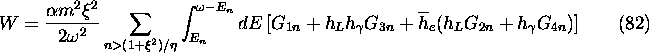, E
Energy of the initial photon and final electron.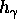,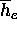Initial photon helicity and `detector helicity' of the final electron.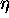Laser energy parameter: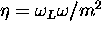(: laser photon energy).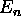Minimum energy of the final electron for given n: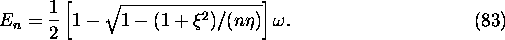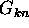Functions involving Bessel functions. See Sec.A.2 for the definition.

When NPH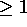is specified in LASERQED command, the above formulas are used with the terms upto n=NPH. See Sec.A for the algorithm of the event generation.Next: Beamstrahlung Up: Laser Previous: Linear Compton Scattering

Toshiaki Tauchi
Thu Dec 3 17:27:26 JST 1998